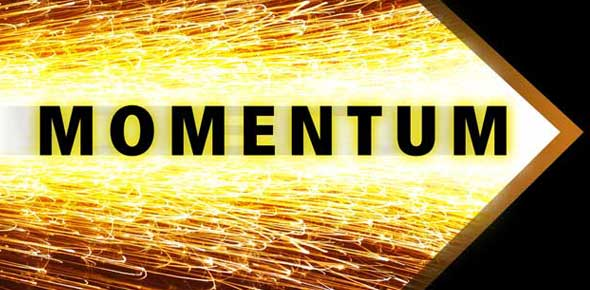# Take The Physics Momentum Quiz Questions!

17 Questions | Attempts: 238
ShareSettings• 1.
Which of the following probably has the largest momentum?
• A.

SUV speeding down the highway

• B.

Santa sliding slowly down the chimney

• C.

Centennial gym

• D.

Mack truck parked on the side of the road

• 2.
It is correct ot say that impulse is equal to
• A.

Momentum

• B.

The force multiplied by the distance the force acts

• C.

The change in momentum

• D.

All of these

• 3.
A cannon fires a 40.5kg shell toward a target and the shell moves with a velocity of 120m/s.  Calculate the shell's momentum.
• A.

4,860 kg x m/s

• B.

4.86 kg x m/s

• C.

2.45 kg x m/s

• D.

10 kg x m/s

• E.

2,500 kg x m/s

• 4.
Calculate the momentum of a 700g ball that is rolling down a ramp at 4.6m/s.
• A.

3.22 kg x m/s

• B.

3,220 kg x m/s

• C.

152.17 kg x m/s

• D.

6.57 kg x m/s

• 5.
Which has greater momentum, a 2.0kg hockey puck moving east at 2.5m/s or a 1.3kg hockey puck moving south at 3.0m/s?
• A.

Hockey puck moving east

• B.

Hockey puck moving south

• C.

They have the same momentum

• 6.
When an object speeds up, it increases it's momentum
• A.

True

• B.

False

• 7.
Objects with different masses can't have the same momentum.
• A.

True

• B.

False

• 8.
A bowling ball has 9 kg m/s of momentum when it is rolled 6 m/s.  What is the mass of the bowling ball?
• A.

54 kg

• B.

15 kg

• C.

3 kg

• D.

1.5 kg

• 9.
Momentum has
• A.

Magnitude only

• B.

Direction only

• C.

Magnitude and direction

• D.

Force and Velocity

• 10.
A bumper car (mass=100 kg) is moving forward with a velocity of 6 m/s. It rebounds off the wall with a velocity (going backward) of 5 m/s. What is the impulse on the car?
• A.

-100 kg m/s

• B.

100 kg m/s

• C.

-1100 kg m/s

• D.

-3000 kg m/s

• 11.
What is momentum?
• A.

Any situation where direction matters

• B.

Energy

• C.

Mass in motion

• D.

A variable measured in Joules

• 12.
How does an airbag protect you?
• A.

It decreases the impulse you experiance

• B.

It decreases the change in momentum you experiance

• C.

It increases the time of impact, hence decreasing the force

• D.

It decrease the time of impact, hence decreasing the force

• 13.
A cheetah (mass=80 kg) is running across the vast Savannah. She starts out with a velocity of 5 m/s and speeds up to 10m/s. What was the impulse acting on the cheetah?
• A.

80 kg m/s

• B.

200 kg m/s

• C.

400 kg m/s

• D.

124 kg m/s

• 14.
A bumper car (mass=100 kg) is moving forward with a velocity of 6 m/s. It rebounds off the wall with a velocity (going backward) of 5 m/s. What is the change in velocity?
• A.

-1 m/s

• B.

1 m/s

• C.

-11 m/s

• D.

-30 m/s

• 15.
A bumper car (mass=100 kg) is moving forward with a velocity of 6 m/s. It rebounds off the wall with a velocity (going backward) of 5 m/s. What is the impulse on the car?
• A.

-100 kg m/s

• B.

100 kg m/s

• C.

-1100 kg m/s

• D.

-3000 kg m/s

• 16.
A bumper car (mass=100 kg) is moving forward with a velocity of 6 m/s. It rebounds off the wall with a velocity (going backward) of 5 m/s. The car is touching the wall for 0.5 seconds. What is the impulse on the car?
• A.

-2200 N

• B.

-200 N

• C.

-6000 N

• D.

200 N

• 17.
You have a mass of 65 kg. You are sitting (at rest) at a stoplight in your car when another car rear ends you. You experience a force of 200 N for 0.5 seconds. What is your final velocity?
• A.

6 m/s

• B.

1.5 m/s

• C.

10 m/s

• D.

8.7 m/s

## Related TopicsBack to top
×

Wait!
Here's an interesting quiz for you.